﻿ On the k-Fibonacci-Like Numbers

### On the k-Fibonacci-Like Numbers

Yashwant K. Panwar, G. P. S. Rathore, Richa ChawlaOPEN ACCESSPEER-REVIEWED

## On the k-Fibonacci-Like Numbers

Yashwant K. Panwar1,, G. P. S. Rathore2, Richa Chawla3

1Department of Mathematics and MCA, Mandsaur Institute of Technology, Mandsaur, India

2Department of Mathematical Sciences, College of Horticulture, Mandsaur, India

3School of Studies in Mathematics, Vikram University, Ujjain, India

### Abstract

The Fibonacci number is famous for possessing wonderful and amazing properties. In this study, we introduce the k-Fibonacci-Like number and related identities. We establish some of the interesting properties of k-Fibonacci-Like number. We shall use the Induction method and Binet’s formula for derivation.

• Panwar, Yashwant K., G. P. S. Rathore, and Richa Chawla. "On the k-Fibonacci-Like Numbers." Turkish Journal of Analysis and Number Theory 2.1 (2014): 9-12.
• Panwar, Y. K. , Rathore, G. P. S. , & Chawla, R. (2014). On the k-Fibonacci-Like Numbers. Turkish Journal of Analysis and Number Theory, 2(1), 9-12.
• Panwar, Yashwant K., G. P. S. Rathore, and Richa Chawla. "On the k-Fibonacci-Like Numbers." Turkish Journal of Analysis and Number Theory 2, no. 1 (2014): 9-12.

 Import into BibTeX Import into EndNote Import into RefMan Import into RefWorks

### 1. Introduction

Many authors have generalized second order recurrence sequences by preserving the recurrence relation and alternating the first two terms of the sequence and some authors have generalized these sequences by preserving the first two terms of the sequence but altering the recurrence relation slightly.

Kalman and Mena  generalize the Fibonacci sequence by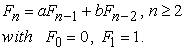(1.1)

Horadam  defined generalized Fibonacci sequence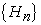by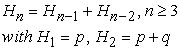(1.2)

where p and q are arbitrary integers.

Singh, Sikhwal, and Bhatnagar , defined Fibonacci-Like sequence by recurrence relation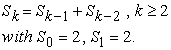(1.3)

The associated initial conditions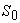and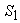are the sum of the Fibonacci and Lucas sequences respectively, i.e.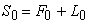and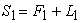.

Natividad , Deriving a Formula in solving Fibonacci-Like sequence. He found missing terms in Fibonacci-Like sequence and solved by standard formula.

Gupta, Panwar and Sikhwal , defined generalized Fibonacci sequences and derived its identities connection formulae and other results. Gupta, Panwar and N. Gupta , stated and derived identities for Fibonacci-Like sequence. Also described and derived connection formulae and negation formula for Fibonacci-Like sequence. Singh, Gupta and Panwar , present many Combinations of Higher Powers of Fibonacci-Like sequence.

The k-Fibonacci numbers defined by Falco’n and Plaza , depending only on one integer parameter k as follows, For any positive real number k, the k-Fibonacci sequence is defined recurrently by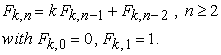(1.4)

Many of the properties of these sequences are proved by simple matrix algebra. This study has been motivated by the arising of two complex valued maps to represent the two antecedents in a specific four-triangle partition. In , Falcon and Plaza k- Fibonacci sequence generalizes, between others, both the classical Fibonacci sequence and the Pell sequence. In this paper many properties of these numbers are deduced and related with the so-called Pascal 2-triangle. New generalized k-Fibonacci sequences have been introduced and studied. Several properties of these numbers are deduced and related with the so-called Pascal 2-triangle. In addition, the generating functions for these k-Fibonacci sequences have been given.

In this paper, we introduced the k-Fibonacci-Like sequence. Also we establish some of the interesting properties of k-Fibonacci-Like numbers like Catalan’s identity, Cassini’s identity, d’ocagnes’s Identity, Binet’s formula and Generating function.

### 2. The k-Fibonacci-Like Sequence

Definition: For any positive real number k, the k-Fibonacci-Like sequenceis defined by for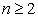,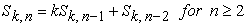(2.1)

The first few k-Fibonacci-Like numbers are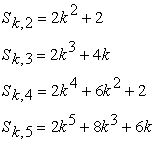Particular case of k-Fibonacci-Like number

If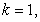k-Fibonacci-Like sequence is obtained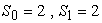and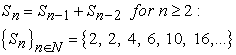### 3. Properties of k-Fibonacci-Like Numbers

3.1. First Explicit Formula for k-Fibonacci-Like Numbers

In the 19th century, the French mathematician Binet devised two remarkable analytical formulas for the Fibonacci and Lucas numbers. In our case, Binet’s formula allows us to express the k-Fibonacci-Like numbers in function of the roots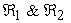of the following characteristic equation, associated to the recurrence relation (2.1)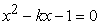(3.1)

Theorem 1: (Binet’s formula). The nth k-Fibonacci-Like number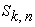is given by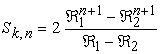(3.2)

where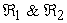are the roots of the characteristic equation (3.1) and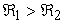.

Proof: We use the Principle of Mathematical Induction (PMI) on n. It is clear the result is true for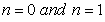by hypothesis. Assume that it is true for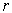such that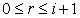, then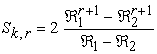It follows from definition of k-generalized Fibonacci numbers (2.1) and equation (3.2)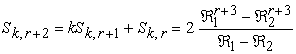Thus, the formula is true for any positive integer n.

where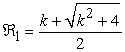and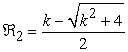.

This completes the proof.

3.2. Catalan's Identity

Catalan's identity for Fibonacci numbers was found in 1879 by Eugene Charles Catalan a Belgian mathematician who worked for the Belgian Academy of Science in the field of number theory.

Theorem 2: (Catalan’s identity)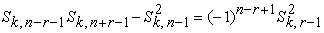(3.3)

Proof: By Binet’s formula (3.2), we have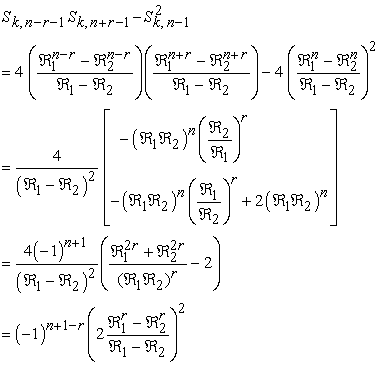This completes the Proof.

3.3. Cassini's Identity

This is one of the oldest identities involving the Fibonacci numbers. It was discovered in 1680 by Jean-Dominique Cassini a French astronomer.

Theorem 3: (Cassini’s identity or Simpson’s identity)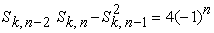(3.4)

Proof. Taking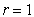in Catalan’s identity (3.3) the proof is completed.

In a similar way that before the following identity is proven:

3.4. d'Ocagne's Identity

Theorem 4: (d’ocagnes’s Identity) If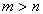then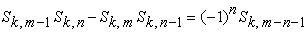(3.5)
3.5. Limit of the Quotient of Two Consecutive Terms

A useful property in these sequences is that the limit of the quotient of two consecutive terms is equal to the positive root of the corresponding characteristic equation

Theorem 5: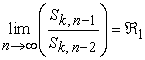(3.6)

Proof. By Binet’s formula (3.2), we have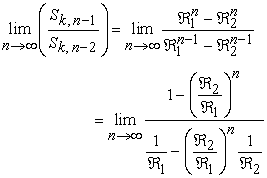and taking into account that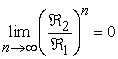, since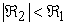, Eq. (3.6) is obtained.

Theorem 6: If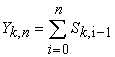, then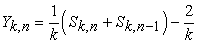(3.7)

Proof. By Binet’s formula (3.2), we have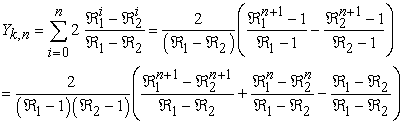This completes the Proof.

Proposition 7: For any integer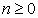,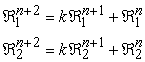(3.8)

Theorem 8: For any integer,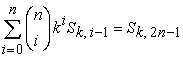(3.9)

Proof . By Binet’s formula (3.2), we have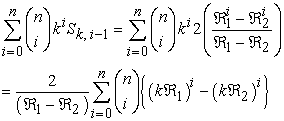By summing up the geometric partial sums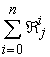for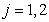. We obtain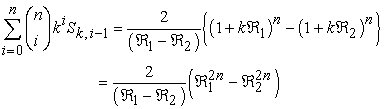This completes the Proof.

3.6. Generating Function for k-Fibonacci-Like Sequence:

Generating functions provide a powerful technique for solving linear homogeneous recurrence relations. Even though generating functions are typically used in conjunction with linear recurrence relations with constant coefficients, we will systematically make use of them for linear recurrence relations with non constant coefficients. In this paragraph, the generating function for k-Fibonacci-Like sequence is given. As a result, k- Fibonacci-Like sequence is seen as the coefficients of the corresponding generating function. Function defined in such a way is called the generating function of the k- Fibonacci-Like sequence. So,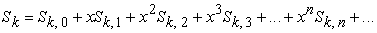and then,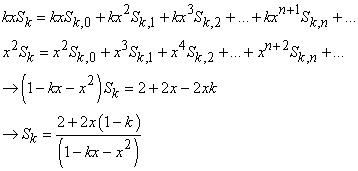(3.10)

### 4. Conclusion

In this paper, k-Fibonacci pattern based sequence introduced which is known as k-Fibonacci-Like sequence.

Many of the properties of this sequence are proved by simple algebra and Binet’s formula. Finally we present properties like Catalan’s identity, Cassini’s identity or Simpson’s identity and d’ocagnes’s identity for k-Fibonacci-Like numbers. Further generating function of k-Fibonacci-Like sequence is presented.

### References

  A. F. Horadam, Basic Properties of Certain Generalized Sequence of Numbers, The Fibonacci Quarterly, 3(3) (1965), 161-176.In article  A. F. Horadam, The Generalized Fibonacci Sequences, The American Math. Monthly, 68(5) (1961), 455-459.In article CrossRef  A. J. Macfarlane, Use of Determinants to present identities involving Fibonacci and Related Numbers, The Fibonacci Quarterly, 48(1) (2010), 68-76.In article  A. T. Benjamin and J. J. Quinn, Recounting Fibonacci and Lucas identities, College Math. J., 30(5) (1999), 359-366.In article CrossRef  B. Singh, O. Sikhwal, and S. Bhatnagar, Fibonacci-Like Sequence and its Properties, Int. J. Contemp. Math. Sciences, 5(18) (2010), 859-868.In article  B. Singh, V. K. Gupta, and Y. K. Panwar, On Combinations of Higher Powers of Fibonacci-Like sequence, Open Journal of Mathematical Modeling, 1 (2), (2013), 63-66.In article CrossRef  D. Kalman and R. Mena, The Fibonacci Numbers – Exposed, The Mathematical Magazine, 2 (2002).In article  L. A. G. Dresel, Transformations of Fibonacci-Lucas identities, Applications of Fibonacci Numbers, 5 (1993), 169-184.In article CrossRef  L. R. Natividad, Deriving a Formula in Solving Fibonacci-like sequence, International Journal of Mathematics and Scientific Computing, 1(1) (2011), 19-21.In article  N. N. Vorobyov, The Fibonacci numbers, D. C. Health and company, Boston, 1963.In article  S. Falco´n, On the k-Lucas numbers. International Journal of Contemporary Mathematical Sciences, 6(21) (2011), 1039-1050.In article  S. Falco´n, On the Lucas Triangle and its Relationship with the k-Lucas numbers. Journal of Mathematical and Computational Science, 2(3) (2012), 425-434.In article  S. Falco´n, Plaza, A.: On the Fibonacci k-numbers. Chaos, Solitons & Fractals, 32(5) (2007), 1615-1624.In article CrossRef  S. Falco´n, Plaza, A.: The k-Fibonacci hyperbolic functions. Chaos, Solitons & Fractals, 38(2) (2008), 409–420.In article CrossRef  S. Falco´n, Plaza, A.: The k-Fibonacci sequence and the Pascal 2-triangle. Chaos, Solitons &Fractals, 33(1) (2007), 38-49.In article CrossRef  S. Vajda, Fibonacci and Lucas numbers, and the golden section. Theory and applications. Chichester: Ellis Horwood limited (1989).In article  T. Koshy, Fibonacci and Lucas numbers with Applications, Wiley, 2001.In article CrossRef  V. K. Gupta, Y. K. Panwar and N. Gupta, identities of Fibonacci-Like sequence, J. Math. Comput. Sci. 2(6) (2012), 1801-1807.In article  V. K. Gupta, Y. K. Panwar and O. Sikhwal, Generalized Fibonacci Sequences, Theoretical Mathematics & Applications, 2(2) (2012), 115-124.In article